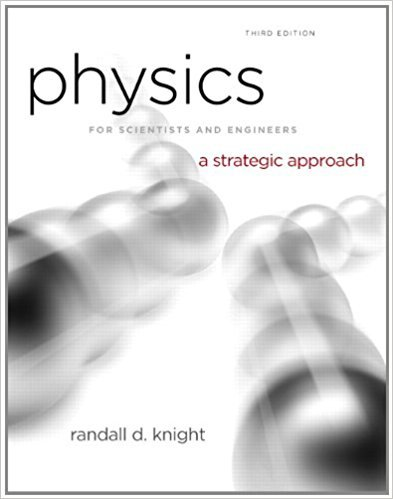×
Get Full Access to Physics For Scientists And Engineers: A Strategic Approach With Modern Physics - 3 Edition - Chapter 11 - Problem 39p
Get Full Access to Physics For Scientists And Engineers: A Strategic Approach With Modern Physics - 3 Edition - Chapter 11 - Problem 39p

×

# A 10 g particle has the potential energy shown inISBN: 9780321740908 69

## Solution for problem 39P Chapter 11

Physics for Scientists and Engineers: A Strategic Approach with Modern Physics | 3rd Edition

• Textbook Solutions
• 2901 Step-by-step solutions solved by professors and subject experts
• Get 24/7 help from StudySoup virtual teaching assistantsPhysics for Scientists and Engineers: A Strategic Approach with Modern Physics | 3rd Edition

4 5 1 241 Reviews
24
4
Problem 39P

Problem 39P

A 10 g particle has the potential energy shown in FIGURE.

a. Draw a force-versus-position graph from x = 0 cm to x =8 cm.

b. How much work does the force do as the particle moves from x =2cm to x =6 cm?

c. What speed does the particle need at x =2cm to arrive at x = 6 cm with a speed of 10 m/s?

FIGUREStep-by-Step Solution:

Solution 39P

Step 1 of 4

(a)

From the given potential energy graph for a particle with mass, we need to draw force versus position graph.

• To derive relation between force and potential energy,

From work energy theorem, we know that work done during the process will be equal to the negative of change in potential energy.

That is,Using work done as product of force and displacement……...1

Step 2 of 4

Step 3 of 4

##### ISBN: 9780321740908

Unlock Textbook Solution